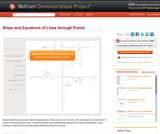# 1 Result

View
Selected filters:
• Tom FalconeConditional Remix & Share Permitted
CC BY-NC-SA
Rating

Algebra students need practice determining equations of lines given a pair of points, or the line parallel or perpendicular to a given line through a given point. This Demonstration, along with guiding worksheets or a teacher presentation, gives students a chance to see the relationships between these lines and points.

Material Type:
Activity/Lab
Diagram/Illustration
Homework/Assignment
Interactive
Simulation
Teaching/Learning Strategy
Provider:
Wolfram Research
Provider Set:
Wolfram Demonstrations Project
Author:
Tom Falcone